• 每一个文件都有自己的文件指纹（MD5值等等相当shenfen证），很多平台会检测文件是否重复（如：1688的详情页图片是否原创检测、百度图片原创筛选、各大视频平台去重等等，他们机器检测MD5发现有与数据库中重复的轻则...
• 可一键批量修改文件MD5特征 防止被和谐或者特征！
• 好用的MD5自动修改器，抓鸡时配合使用，防止MD5值被杀，抓鸡神器！
• 文件MD5修改器是一款专门用于修改文件MD5数值的小工具。MD5在以前是文件唯一的身份ID，类似于我们的身份证号码一样是唯一的的。但是随着网络的发展已经有人破解出算法。所以我们现在下载文件，也不能只相信MD5了。...
• 基础方法:打开md5破解,exe输入MD5值，按回车即可 高级方法:用命令行进入md5破解所在目录执行东方黒盟md5破解 <MD5值> <配置文件名> 注意事项:请将本程序放在英文路径下,放在中文路径下可能会出现问题 注意：破解的...
• MD5可以为任何文件（不管其大小、格式、数量）产生一个同样独一无二的"数字指纹"，如果任何人对文件做了任何改动，其MD5值也就是对应的"数字指纹"都会发生变化。可以通过修改软件的MD5值，躲避杀软的监控.
• 修改文件MD5值，方便上传小视频。修改文件MD5值，方便上传小视频。
• CRC32: 812B5F0B MD5: 083D2F9752C69339E7B9EC8BCED0AB69 SHA-1: 9FAAFAA5CD8F99E829CA...3、一键修改文件或文件夹内文件md5 4、增加托盘图标 5、优化了修改流程，使修改速度更快 2011-8-17 18:30:59 发布 火眼查毒： ...
• MD5校验工具是用来校验下载的资源是否与官网提供的资源一直，如果校验出来的MD5值与官方提供的MD5值不一致，则说明你下载的文件被修改过，请谨慎使用
• ●该程序能将fbx动画导出md5骨骼动画,封装的exe程序(非max脚本,非插件). ●输入的fbx和输出的md5坐标系都是采用Z朝上的max坐标(标准Md5坐标系). ●输入的fbx路径和文件名只能是英文,输出路径可为中文. ●数据算法...
• wampserver服务器用于搭建自己的服务器系统，参数可配，简单易用服务器
• ## 修改文件的MD5

千次阅读 2019-03-14 19:40:55
原标题是：修改文件MD5 原作者是：筝风放风筝 原文链接是： https://blog.csdn.net/Feng512275/article/details/81431467 这篇文章复制的方法是：在浏览器中审查元素（点F12），复制HTML元素，直接粘贴在此处。 ...
原文链接： https://blog.csdn.net/Feng512275/article/details/81431467 本文转载方法：在浏览器中审查元素(F12)，复制HTML元素，直接粘贴在此处。 详见教程：https://blog.csdn.net/qq_34646449/article/details/73844023
本文亲测有效！上传 Teacher Cang 的视频到某知名限速网盘，会成为一个“净网公告”， 按本文操作，修改文件的MD5，就可以上传了。

背景
文件的MD5就像文件的ID一样，唯一标识一个文件。
MD是Message Digest的缩写，翻译为信息摘要或数字签名。MD5是散列\哈希算法的一种，其他的算法还有SHA系列算法，这种算法能通过文件的信息计算出的一组数字，来唯一标识这个文件，而且是不可逆的，但是一旦文件被篡改，哪怕是一点点，计算出的信息摘要就不一样了。
某些网站会提供下载软件的MD5，你可以自己通过MD5算法计算并进行对比确定软件有无篡改
你要发某文本给别人，可以将文本内容MD5计算信息摘要，再将文本内容用你的私钥加密，向别人发送私钥加密文本和MD5摘要，别人得到后，用你的公钥解密文本，自己计算MD5并进行对比，如果一样，就说明没有被篡改。

MD5的应用
那么MD5有什么应用呢？
某盘不是经常河蟹一些视频吗？ 那他是怎么做到的呢？ 他不可能所有的视频都看一遍吧，这样太耗费人力了。（当然，也有鉴黄师人工操作的，但毕竟大批量的还是交给电脑来处理的） 其实就是保存了各个视频的MD5而已，你上传的东西，对比一下他保存的MD5是否一样，如果一样，那简单，直接和谐好了。
em…清楚了这个原理，那么我就可以搞一波骚操作了，我修改他的MD5，生成一个新的MD5，那某盘就没法识别了。

修改MD5
打开CMD命令行，然后进入你要修改的文件所在的文件夹。
命令：CD xxxx对应的文件夹xx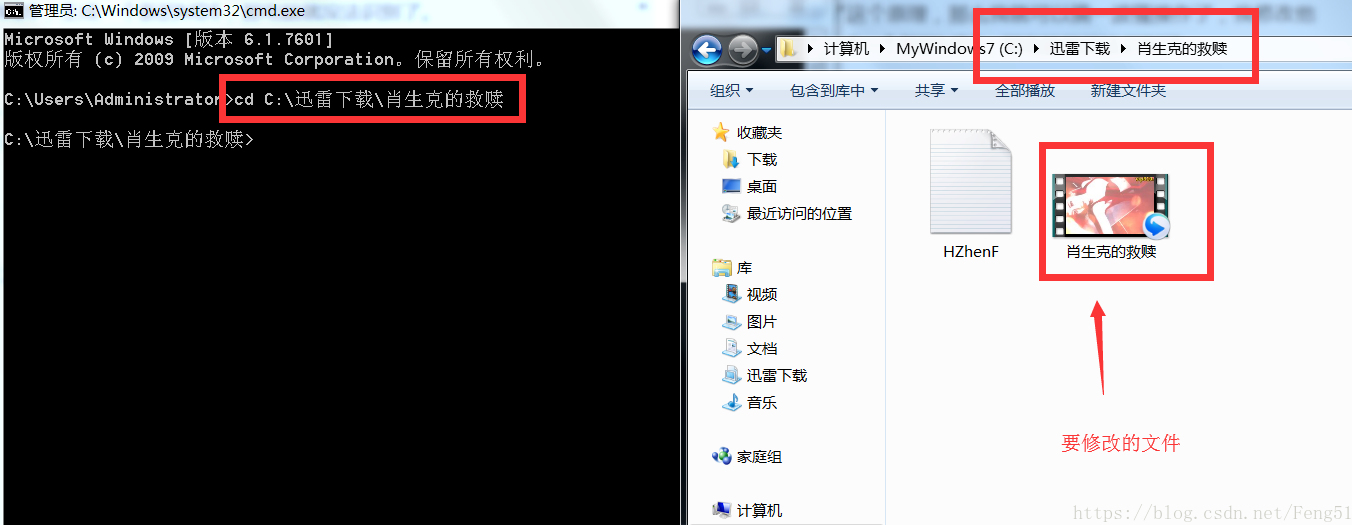创建一个新的记事本(名字随便起，上图中我创建的记事本命名为“HZhenF”，内容随便输入一点就好) 用原视频+HZhenF记事本生成一个新的MD5视频
格式：copy /b 原视频+txt文件名 新的视频名字 命令：copy /b 肖生克的救赎.mp4+HZhenF.txt 肖生克的救赎_新文件.mp4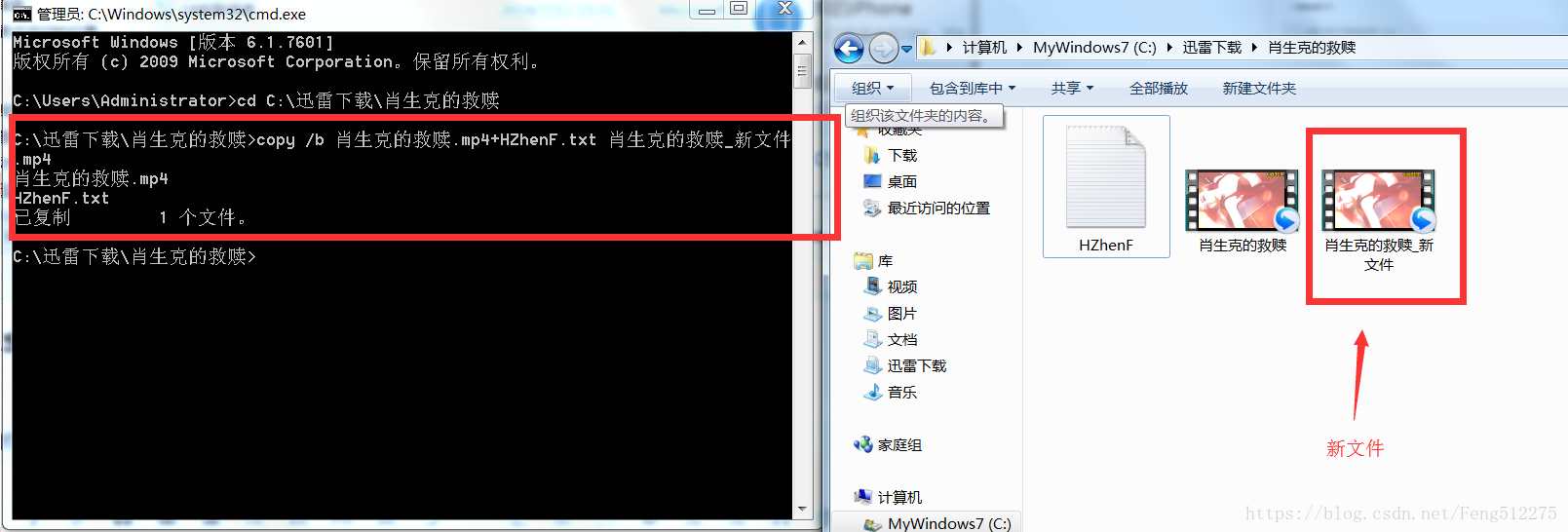查看文件的MD5 命令：certutil -hashfile 文件名.后缀 MD5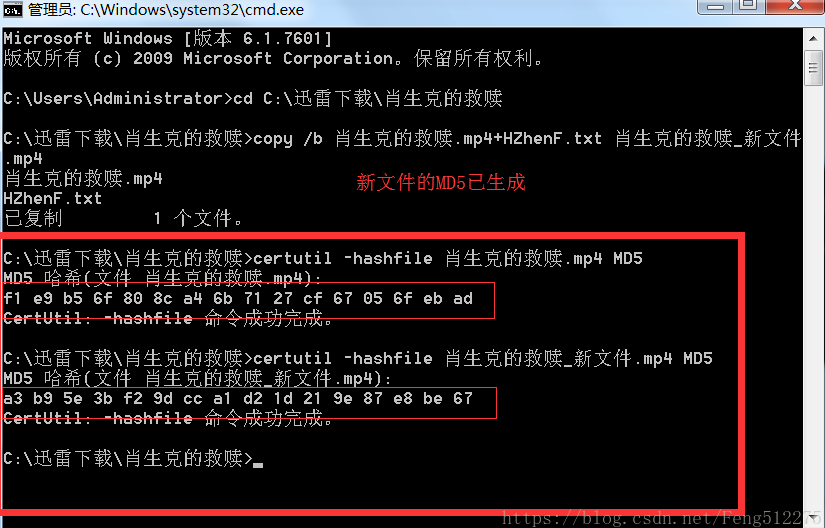展开全文• md5的方式，对文件进行加密处理，其中生成是md5值，是判断文件是否被修改的重要凭证。

使用MD5的方式对文件进行加密，判断获取文件是否被修改的唯一凭证：MD5值

public class TestFileMD5 {            public final static String[] hexDigits = { "0", "1", "2", "3", "4", "5",          "6", "7", "8", "9", "a", "b", "c", "d", "e", "f" };            /**      * 获取文件的MD5值      * @param file      * @return      */      public static String getFileMD5(File file){          String md5 = null;          FileInputStream fis = null;          FileChannel fileChannel = null;          try {              fis = new FileInputStream(file);              fileChannel = fis.getChannel();              MappedByteBuffer byteBuffer = fileChannel.map(FileChannel.MapMode.READ_ONLY, 0, file.length());                            try {                  MessageDigest md = MessageDigest.getInstance("MD5");                  md.update(byteBuffer);                  md5 = byteArrayToHexString(md.digest());              } catch (NoSuchAlgorithmException e) {                                    e.printStackTrace();              }          } catch (FileNotFoundException e) {                            e.printStackTrace();          } catch (IOException e) {                            e.printStackTrace();          }finally{              try {                  fileChannel.close();                  fis.close();              } catch (IOException e) {                                    e.printStackTrace();              }          }                    return md5;      }            /**      * 字节数组转十六进制字符串      * @param digest      * @return      */      private static String byteArrayToHexString(byte[] digest) {                    StringBuffer buffer = new StringBuffer();          for(int i=0; i<digest.length; i++){              buffer.append(byteToHexString(digest[i]));          }          return buffer.toString();      }            /**      * 字节转十六进制字符串      * @param b      * @return      */      private static String byteToHexString(byte b) {          //  int d1 = n/16;                int d1 = (b&0xf0)>>4;                         //   int d2 = n%16;                int d2 = b&0xf;               return hexDigits[d1] + hexDigits[d2];      }            //入口       public static void main(String [] args) throws Exception{          System.out.println("-----测试创建文件的md5后缀----------");                    File file = new File("E:/jquery-1.9.1.min.js");                    if(!file.exists()){              file.createNewFile();          }          //获取参数           String parent = file.getParent();                    System.out.println(parent);          String fileName = file.getName();          System.out.println(fileName);          //首先获取文件的MD5           String md5 = getFileMD5(file);                    //93d97357be249c61407fa21aa434e72f           System.out.println("-----获取的md5：" + md5);                    //组装           File md5File = new File(parent + fileName +".md5");          if(md5File.exists()){              md5File.delete();              md5File.createNewFile();          }                    FileOutputStream fos = new FileOutputStream(md5File);          fos.write(md5.getBytes());                    fos.flush();          fos.close();                    System.out.println("--------完成---------");      }  }

注意：

使用md5的方式对文件进行加密，以获取md5值，可以知道该文件的内容是否被修改过，

因为修改过文件内容，再次对该文件进行加密的话，获取的md5值将发生变化。


展开全文• 适用于一些md5验证的场合，每次处理之后都生成随机的md5验证码，响应速度快，可批量修改。处理之后不影响文件的使用。
• 拖拽文件或文件夹至getMD5.exe上，可自动计算文件或文件夹下所有文件的MD5值，保存至文件MD5.txt中 方式三： 通过cmd命令调用（类似方式二） REM 获取1.txt和files目录下所有文件的MD5值 getMD5.exe &...
方式一：
双击运行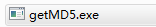，输入字符串计算MD5值；或拖动任意文件或文件夹到到工具上，自动计算MD5值。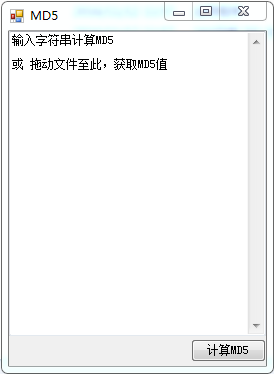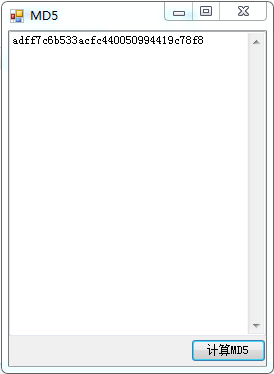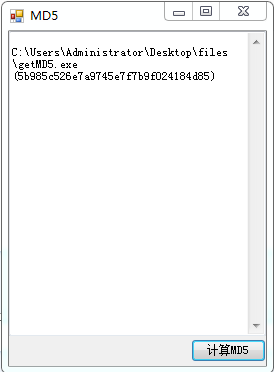方式二：
拖拽文件或文件夹至getMD5.exe上，可自动计算文件或文件夹下所有文件的MD5值，保存至文件MD5.txt中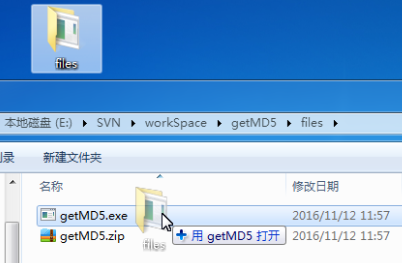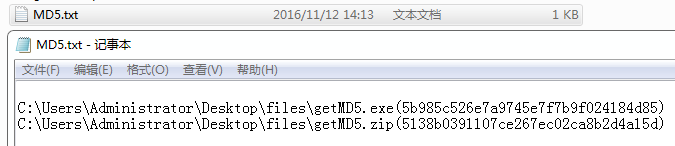方式三：
通过cmd命令调用（类似方式二）

REM 获取1.txt和files目录下所有文件的MD5值
getMD5.exe "D:\tmp\1.txt;C:\Users\Administrator\Desktop\files"

MD5计算工具下载

工具源码：

using System;
using System.Collections.Generic;
using System.IO;
using System.Linq;
using System.Text;

namespace getMD5
{
//示例：
// MD5.Encrypt("a");                        // 计算字符串MD5值
// MD5.Encrypt(new FileInfo("D:\\1.rar"));  // 计算文件MD5值
// MD5.Encrypt(byte[] Bytes);               // 计算Byte数组MD5值

//MD5 ("") = d41d8cd98f00b204e9800998ecf8427e
//MD5 ("a") = 0cc175b9c0f1b6a831c399e269772661
//MD5 ("abc") = 900150983cd24fb0d6963f7d28e17f72
//MD5 ("message digest") = f96b697d7cb7938d525a2f31aaf161d0
//MD5 ("abcdefghijklmnopqrstuvwxyz") = c3fcd3d76192e4007dfb496cca67e13b
class MD5
{

#region MD5调用接口

/// <summary>
/// 计算data的MD5值
/// </summary>
public static string Encrypt(string data)
{
uint[] X = To16Array(data);
return calculate(X);
}

/// <summary>
/// 计算byte数组的MD5值
/// </summary>
public static string Encrypt(byte[] Bytes)
{
uint[] X = To16Array(Bytes);
return calculate(X);
}

/// <summary>
/// 计算文件的MD5值
/// </summary>
public static string Encrypt(FileInfo file)
{
uint[] X = To16Array(file);
return calculate(X);
}

#endregion

#region MD5计算逻辑

/// <summary>
/// 转化byte数组为uint数组，数组长度为16的倍数
///
/// 1、字符串转化为字节数组，每4个字节转化为一个uint，依次存储到uint数组
/// 2、附加0x80作为最后一个字节
/// 3、在uint数组最后位置记录文件字节长度信息
/// </summary>
public static uint[] To16Array(byte[] Bytes)
{
uint DataLen = (uint)Bytes.Length;

// 计算FileLen对应的uint长度（要求为16的倍数、预留2个uint、最小为16）
uint ArrayLen = (((DataLen + 8) / 64) + 1) * 16;
uint[] Array = new uint[ArrayLen];

uint ArrayPos = 0;
int pos = 0;
uint ByteCount = 0;
for (ByteCount = 0; ByteCount < DataLen; ByteCount++)
{
// 每4个Byte转化为1个uint
ArrayPos = ByteCount / 4;
pos = (int)(ByteCount % 4) * 8;
Array[ArrayPos] = Array[ArrayPos] | ((uint)Bytes[ByteCount] << pos);
}

// 附加0x80作为最后一个字节，添加到uint数组对应位置
ArrayPos = ByteCount / 4;
pos = (int)(ByteCount % 4) * 8;
Array[ArrayPos] = Array[ArrayPos] | ((uint)0x80 << pos);

// 记录总长度信息
Array[ArrayLen - 2] = (DataLen << 3);
Array[ArrayLen - 1] = (DataLen >> 29);

return Array;
}

/// <summary>
/// 转化字符串为uint数组，数组长度为16的倍数
///
/// 1、字符串转化为字节数组，每4个字节转化为一个uint，依次存储到uint数组
/// 2、附加0x80作为最后一个字节
/// 3、在uint数组最后位置记录文件字节长度信息
/// </summary>
public static uint[] To16Array(string data)
{
byte[] datas = System.Text.Encoding.Default.GetBytes(data);
}

/// <summary>
/// 转化文件为uint数组，数组长度为16的倍数
///
/// 1、读取文件字节信息，每4个字节转化为一个uint，依次存储到uint数组
/// 2、附加0x80作为最后一个字节
/// 3、在uint数组最后位置记录文件字节长度信息
/// </summary>
public static uint[] To16Array(FileInfo info)
{
FileStream fs = new FileStream(info.FullName, FileMode.Open);// 读取方式打开，得到流
int SIZE = 1024 * 1024 * 10;        // 10M缓存
byte[] datas = new byte[SIZE];      // 要读取的内容会放到这个数组里
int countI = 0;
long offset = 0;

// 计算FileLen对应的uint长度（要求为16的倍数、预留2个uint、最小为16）
uint FileLen = (uint)info.Length;
uint ArrayLen = (((FileLen + 8) / 64) + 1) * 16;
uint[] Array = new uint[ArrayLen];

int pos = 0;
uint ByteCount = 0;
uint ArrayPos = 0;
while (ByteCount < FileLen)
{
if (countI == 0)
{
fs.Seek(offset, SeekOrigin.Begin);// 定位到指定字节

offset += SIZE;
}

// 每4个Byte转化为1个uint
ArrayPos = ByteCount / 4;
pos = (int)(ByteCount % 4) * 8;
Array[ArrayPos] = Array[ArrayPos] | ((uint)datas[countI] << pos);

ByteCount = ByteCount + 1;

countI++;
if (countI == SIZE) countI = 0;
}

// 附加0x80作为最后一个字节，添加到uint数组对应位置
ArrayPos = ByteCount / 4;
pos = (int)(ByteCount % 4) * 8;
Array[ArrayPos] = Array[ArrayPos] | ((uint)0x80 << pos);

// 记录总长度信息
Array[ArrayLen - 2] = (FileLen<< 3);
Array[ArrayLen - 1] = (FileLen>>29);

fs.Close();
return Array;
}

private static uint F(uint x, uint y, uint z)
{
return (x & y) | ((~x) & z);
}
private static uint G(uint x, uint y, uint z)
{
return (x & z) | (y & (~z));
}

// 0^0^0 = 0
// 0^0^1 = 1
// 0^1^0 = 1
// 0^1^1 = 0
// 1^0^0 = 1
// 1^0^1 = 0
// 1^1^0 = 0
// 1^1^1 = 1
private static uint H(uint x, uint y, uint z)
{
return (x ^ y ^ z);
}
private static uint I(uint x, uint y, uint z)
{
return (y ^ (x | (~z)));
}

// 循环左移
private static uint RL(uint x, int y)
{
y = y % 32;
return (x << y) | (x >> (32 - y));
}

private static void md5_FF(ref uint a, uint b, uint c, uint d, uint x, int s, uint ac)
{
uint f = F(b, c, d);
a = x + ac + a + f;

a = RL(a, s);
a = a + b;
}
private static void md5_GG(ref uint a, uint b, uint c, uint d, uint x, int s, uint ac)
{
uint g = G(b, c, d);
a = x + ac + a + g;

a = RL(a, s);
a = a + b;
}
private static void md5_HH(ref uint a, uint b, uint c, uint d, uint x, int s, uint ac)
{
uint h = H(b, c, d);
a = x + ac + a + h;

a = RL(a, s);
a = a + b;
}
private static void md5_II(ref uint a, uint b, uint c, uint d, uint x, int s, uint ac)
{
uint i = I(b, c, d);
a = x + ac + a + i;

a = RL(a, s);
a = a + b;
}

private static string RHex(uint n)
{
string S = Convert.ToString(n, 16);
return ReOrder(S);
}

// 16进制串重排序 67452301 -> 01234567
private static string ReOrder(String S)
{
string T = "";
for (int i = S.Length - 2; i >= -1; i = i - 2)
{
if (i == -1) T = T + "0" + S[i + 1];
else T = T + "" + S[i] + S[i + 1];
}
return T;
}

/// <summary>
/// 对长度为16倍数的uint数组，执行md5数据摘要，输出md5信息
/// </summary>
public static string calculate(uint[] x)
{
//uint time1 = DateTime.Now.Ticks;

// 7   12  17   22
// 5   9   14   20
// 4   11  16   23
// 6   10  15   21
const int S11 = 7;
const int S12 = 12;
const int S13 = 17;
const int S14 = 22;
const int S21 = 5;
const int S22 = 9;
const int S23 = 14;
const int S24 = 20;
const int S31 = 4;
const int S32 = 11;
const int S33 = 16;
const int S34 = 23;
const int S41 = 6;
const int S42 = 10;
const int S43 = 15;
const int S44 = 21;

uint a = 0x67452301;
uint b = 0xEFCDAB89;
uint d = 0x10325476;

for (int k = 0; k < x.Length; k += 16)
{
uint AA = a;
uint BB = b;
uint CC = c;
uint DD = d;

md5_FF(ref a, b, c, d, x[k + 0], S11, 0xD76AA478);  // 3604027302
md5_FF(ref d, a, b, c, x[k + 1], S12, 0xE8C7B756);  // 877880356
md5_FF(ref c, d, a, b, x[k + 2], S13, 0x242070DB);  // 2562383102
md5_FF(ref b, c, d, a, x[k + 3], S14, 0xC1BDCEEE);
md5_FF(ref a, b, c, d, x[k + 4], S11, 0xF57C0FAF);
md5_FF(ref d, a, b, c, x[k + 5], S12, 0x4787C62A);
md5_FF(ref c, d, a, b, x[k + 6], S13, 0xA8304613);
md5_FF(ref b, c, d, a, x[k + 7], S14, 0xFD469501);
md5_FF(ref a, b, c, d, x[k + 8], S11, 0x698098D8);
md5_FF(ref d, a, b, c, x[k + 9], S12, 0x8B44F7AF);
md5_FF(ref c, d, a, b, x[k + 10], S13, 0xFFFF5BB1);
md5_FF(ref b, c, d, a, x[k + 11], S14, 0x895CD7BE);
md5_FF(ref a, b, c, d, x[k + 12], S11, 0x6B901122);
md5_FF(ref d, a, b, c, x[k + 13], S12, 0xFD987193);
md5_FF(ref c, d, a, b, x[k + 14], S13, 0xA679438E);
md5_FF(ref b, c, d, a, x[k + 15], S14, 0x49B40821); //3526238649
md5_GG(ref a, b, c, d, x[k + 1], S21, 0xF61E2562);
md5_GG(ref d, a, b, c, x[k + 6], S22, 0xC040B340);  //1572812400
md5_GG(ref c, d, a, b, x[k + 11], S23, 0x265E5A51);
md5_GG(ref b, c, d, a, x[k + 0], S24, 0xE9B6C7AA);
md5_GG(ref a, b, c, d, x[k + 5], S21, 0xD62F105D);
md5_GG(ref d, a, b, c, x[k + 10], S22, 0x2441453);
md5_GG(ref c, d, a, b, x[k + 15], S23, 0xD8A1E681);
md5_GG(ref b, c, d, a, x[k + 4], S24, 0xE7D3FBC8);
md5_GG(ref a, b, c, d, x[k + 9], S21, 0x21E1CDE6);
md5_GG(ref d, a, b, c, x[k + 14], S22, 0xC33707D6);
md5_GG(ref c, d, a, b, x[k + 3], S23, 0xF4D50D87);
md5_GG(ref b, c, d, a, x[k + 8], S24, 0x455A14ED);
md5_GG(ref a, b, c, d, x[k + 13], S21, 0xA9E3E905);
md5_GG(ref d, a, b, c, x[k + 2], S22, 0xFCEFA3F8);
md5_GG(ref c, d, a, b, x[k + 7], S23, 0x676F02D9);
md5_GG(ref b, c, d, a, x[k + 12], S24, 0x8D2A4C8A);
md5_HH(ref a, b, c, d, x[k + 5], S31, 0xFFFA3942);  // 3750198684 2314002400 1089690627 990001115 0 4 -> 2749600077
md5_HH(ref d, a, b, c, x[k + 8], S32, 0x8771F681);  // 990001115
md5_HH(ref c, d, a, b, x[k + 11], S33, 0x6D9D6122); // 1089690627
md5_HH(ref b, c, d, a, x[k + 14], S34, 0xFDE5380C); // 2314002400
md5_HH(ref a, b, c, d, x[k + 1], S31, 0xA4BEEA44);  // 555633090
md5_HH(ref d, a, b, c, x[k + 4], S32, 0x4BDECFA9);
md5_HH(ref c, d, a, b, x[k + 7], S33, 0xF6BB4B60);
md5_HH(ref b, c, d, a, x[k + 10], S34, 0xBEBFBC70);
md5_HH(ref a, b, c, d, x[k + 13], S31, 0x289B7EC6);
md5_HH(ref d, a, b, c, x[k + 0], S32, 0xEAA127FA);
md5_HH(ref c, d, a, b, x[k + 3], S33, 0xD4EF3085);
md5_HH(ref b, c, d, a, x[k + 6], S34, 0x4881D05);
md5_HH(ref a, b, c, d, x[k + 9], S31, 0xD9D4D039);
md5_HH(ref d, a, b, c, x[k + 12], S32, 0xE6DB99E5);
md5_HH(ref c, d, a, b, x[k + 15], S33, 0x1FA27CF8);
md5_HH(ref b, c, d, a, x[k + 2], S34, 0xC4AC5665);  // 1444303940
md5_II(ref a, b, c, d, x[k + 0], S41, 0xF4292244);  // 808311156
md5_II(ref d, a, b, c, x[k + 7], S42, 0x432AFF97);
md5_II(ref c, d, a, b, x[k + 14], S43, 0xAB9423A7);
md5_II(ref b, c, d, a, x[k + 5], S44, 0xFC93A039);
md5_II(ref a, b, c, d, x[k + 12], S41, 0x655B59C3);
md5_II(ref d, a, b, c, x[k + 3], S42, 0x8F0CCC92);
md5_II(ref c, d, a, b, x[k + 10], S43, 0xFFEFF47D);
md5_II(ref b, c, d, a, x[k + 1], S44, 0x85845DD1);
md5_II(ref a, b, c, d, x[k + 8], S41, 0x6FA87E4F);
md5_II(ref d, a, b, c, x[k + 15], S42, 0xFE2CE6E0);
md5_II(ref c, d, a, b, x[k + 6], S43, 0xA3014314);
md5_II(ref b, c, d, a, x[k + 13], S44, 0x4E0811A1);
md5_II(ref a, b, c, d, x[k + 4], S41, 0xF7537E82);
md5_II(ref d, a, b, c, x[k + 11], S42, 0xBD3AF235);
md5_II(ref c, d, a, b, x[k + 2], S43, 0x2AD7D2BB);
md5_II(ref b, c, d, a, x[k + 9], S44, 0xEB86D391);  // 4120542881

a = a + AA; //3844921825
b = b + BB;
c = c + CC;
d = d + DD;
}

string MD5 = RHex(a) + RHex(b) + RHex(c) + RHex(d);

//uint time2 = DateTime.Now.Ticks;
//MessageBox.Show("MD5计算耗时：" + ((time2 - time1) / 10000000f) + "秒");

return MD5;
}

#endregion

}
}


using System;
using System.Collections.Generic;
using System.Linq;
using System.Windows.Forms;

namespace getMD5
{
static class Program
{
/// <summary>
/// 应用程序的主入口点。
/// </summary>
static void Main(string[] args)
{
Application.EnableVisualStyles();
Application.SetCompatibleTextRenderingDefault(false);

if (args.Length == 0) Application.Run(new Form_MD5());
else if (args.Length == 1)
{
string[] files = args.Split(';');
string filesNames = Form_MD5.toSubDirFileNames(files);
files = filesNames.Split(';');

string tmp = Form_MD5.getFilesMD5(files);   // 计算文件MD5值
Form_MD5.SaveProcess(tmp);
}
}
}
}

using System;
using System.Collections.Generic;
using System.ComponentModel;
using System.Data;
using System.Drawing;
using System.Linq;
using System.Text;
using System.Windows.Forms;

namespace getMD5
{
public partial class Form_MD5 : Form
{
public Form_MD5()
{
InitializeComponent();
}

private void button1_Click(object sender, EventArgs e)
{
textBox1.Text = MD5.Encrypt(textBox1.Text, 32);
}

private void Form_DragEnter(object sender, DragEventArgs e)
{
dragEnter(e);
}

private void Form_DragDrop(object sender, DragEventArgs e)
{
TextBox textBox = sender as TextBox;
textBox.Text = dragDrop(e);                 // 获取拖入的文件
string[] files = textBox.Text.Split(';');

string tmp = getFilesMD5(files);            // 计算文件MD5值
textBox1.Text = tmp;
SaveProcess(tmp, "MD5.txt");
}

// 计算各文件对应的MD5值
public static String getFilesMD5(string[] files)
{
string tmp = "";

foreach (string file in files)
{
if (System.IO.File.Exists(file))
{
string data = fileToString(file);
string md5 = MD5.Encrypt(data, 32);
tmp += "\r\n" + file + "(" + md5 + ")";
}
}

return tmp;
}

#region 文件读取与保存

/// <summary>
/// 获取文件中的数据串
/// </summary>
public static string fileToString(String filePath)
{
string str = "";

//获取文件内容
if (System.IO.File.Exists(filePath))
{

file1.Close();
file1.Dispose();
}
return str;
}

/// <summary>
/// 保存数据data到文件处理过程，返回值为保存的文件名
/// </summary>
public static String SaveProcess(String data, String name = "", String CurDir = "")
{
if (CurDir.Equals("")) CurDir = System.AppDomain.CurrentDomain.BaseDirectory;           //设置当前目录
if (name.Equals("")) name = "MD5.txt";
if (!System.IO.Directory.Exists(CurDir)) System.IO.Directory.CreateDirectory(CurDir);   //该路径不存在时，在当前文件目录下创建文件夹"导出.."

//不存在该文件时先创建
String filePath = CurDir + name;
System.IO.StreamWriter file1 = new System.IO.StreamWriter(filePath, false);     //文件已覆盖方式添加内容

file1.Write(data);                                                              //保存数据到文件

file1.Close();                                                                  //关闭文件
file1.Dispose();                                                                //释放对象

return filePath;
}

#endregion

# region 文件拖拽

/// <summary>
/// 文件拖进事件处理：
/// </summary>
public void dragEnter(DragEventArgs e)
{
if (e.Data.GetDataPresent(DataFormats.FileDrop))    //判断拖来的是否是文件
else e.Effect = DragDropEffects.None;
}

/// <summary>
/// 文件放下事件处理：
/// 放下, 另外需设置对应控件的 AllowDrop = true;
/// 获取的文件名形如 "d:\1.txt;d:\2.txt"
/// </summary>
public string dragDrop(DragEventArgs e)
{
Array file = (System.Array)e.Data.GetData(DataFormats.FileDrop);//将拖来的数据转化为数组存储
}

// 获取所有files目录下的所有文件，转化为单个串
public static string toSubDirFileNames(Array files)
{
StringBuilder filesName = new StringBuilder("");

foreach (object I in files)
{
string str = I.ToString();

System.IO.FileInfo info = new System.IO.FileInfo(str);
//若为目录，则获取目录下所有子文件名
if ((info.Attributes & System.IO.FileAttributes.Directory) != 0)
{
str = getAllFiles(str);
if (!str.Equals("")) filesName.Append((filesName.Length == 0 ? "" : ";") + str);
}
//若为文件，则获取文件名
else if (System.IO.File.Exists(str))
filesName.Append((filesName.Length == 0 ? "" : ";") + str);
}

return filesName.ToString();
}

/// <summary>
/// 判断path是否为目录
/// </summary>
public bool IsDirectory(String path)
{
System.IO.FileInfo info = new System.IO.FileInfo(path);
return (info.Attributes & System.IO.FileAttributes.Directory) != 0;
}

/// <summary>
/// 获取目录path下所有子文件名
/// </summary>
public static string getAllFiles(String path)
{
StringBuilder str = new StringBuilder("");
if (System.IO.Directory.Exists(path))
{
//所有子文件名
string[] files = System.IO.Directory.GetFiles(path);
foreach (string file in files)
str.Append((str.Length == 0 ? "" : ";") + file);

//所有子目录名
string[] Dirs = System.IO.Directory.GetDirectories(path);
foreach (string dir in Dirs)
{
string tmp = getAllFiles(dir);  //子目录下所有子文件名
if (!tmp.Equals("")) str.Append((str.Length == 0 ? "" : ";") + tmp);
}
}
return str.ToString();
}

# endregion

}
}

namespace getMD5
{
partial class Form_MD5
{
/// <summary>
/// 必需的设计器变量。
/// </summary>
private System.ComponentModel.IContainer components = null;

/// <summary>
/// 清理所有正在使用的资源。
/// </summary>
/// <param name="disposing">如果应释放托管资源，为 true；否则为 false。</param>
protected override void Dispose(bool disposing)
{
if (disposing && (components != null))
{
components.Dispose();
}
base.Dispose(disposing);
}

#region Windows 窗体设计器生成的代码

/// <summary>
/// 设计器支持所需的方法 - 不要
/// 使用代码编辑器修改此方法的内容。
/// </summary>
private void InitializeComponent()
{
this.components = new System.ComponentModel.Container();
this.button1 = new System.Windows.Forms.Button();
this.textBox1 = new System.Windows.Forms.TextBox();
this.toolTip1 = new System.Windows.Forms.ToolTip(this.components);
this.SuspendLayout();
//
// button1
//
this.button1.Anchor = ((System.Windows.Forms.AnchorStyles)((System.Windows.Forms.AnchorStyles.Bottom | System.Windows.Forms.AnchorStyles.Right)));
this.button1.Location = new System.Drawing.Point(182, 308);
this.button1.Name = "button1";
this.button1.Size = new System.Drawing.Size(75, 23);
this.button1.TabIndex = 0;
this.button1.Text = "计算MD5";
this.button1.UseVisualStyleBackColor = true;
this.button1.Click += new System.EventHandler(this.button1_Click);
//
// textBox1
//
this.textBox1.AllowDrop = true;
this.textBox1.Anchor = ((System.Windows.Forms.AnchorStyles)((((System.Windows.Forms.AnchorStyles.Top | System.Windows.Forms.AnchorStyles.Bottom)
| System.Windows.Forms.AnchorStyles.Left)
| System.Windows.Forms.AnchorStyles.Right)));
this.textBox1.Location = new System.Drawing.Point(0, 0);
this.textBox1.Multiline = true;
this.textBox1.Name = "textBox1";
this.textBox1.ScrollBars = System.Windows.Forms.ScrollBars.Both;
this.textBox1.Size = new System.Drawing.Size(257, 305);
this.textBox1.TabIndex = 1;
this.toolTip1.SetToolTip(this.textBox1, "可拖动文件至此，查看文件的MD5值");
this.textBox1.DragDrop += new System.Windows.Forms.DragEventHandler(this.Form_DragDrop);
this.textBox1.DragEnter += new System.Windows.Forms.DragEventHandler(this.Form_DragEnter);
//
// Form1
//
this.AutoScaleDimensions = new System.Drawing.SizeF(6F, 12F);
this.AutoScaleMode = System.Windows.Forms.AutoScaleMode.Font;
this.ClientSize = new System.Drawing.Size(257, 335);
this.Name = "Form1";
this.Text = "MD5";
this.ResumeLayout(false);
this.PerformLayout();

}

#endregion

private System.Windows.Forms.Button button1;
private System.Windows.Forms.TextBox textBox1;
private System.Windows.Forms.ToolTip toolTip1;
}
}

工具源码下载
开源地址

展开全文• 此工具主要是验证我们下载的软件是否是未经第三方修改的原生软件，通过MD5码可以判断软件是否被修改，本软件（MD5验证工具.exe）文件的MD5为：723dc31579a08ab69f06ff4349b730ee 本工具大家可以免费传播与使用,如...
• ## md5加密原理 MD5简介

千次阅读 2018-11-10 09:54:28
md5加密原理 MD5简介
分享一下我老师大神的人工智能教程！零基础，通俗易懂！http://blog.csdn.net/jiangjunshow

也欢迎大家转载本篇文章。分享知识，造福人民，实现我们中华民族伟大复兴！

http://help.liangjing.org/Tools/MD5.aspx

给我老师的人工智能教程打call！http://blog.csdn.net/jiangjunshow展开全文• 主要功能为：1，计算文件夹下所有文件的MD5值，并输出到MD5_file.txt文件； 2，在不同（也可相同电脑比较不同版本）电脑上，比较该文件夹下的文件是否存在增删改，以及文件名修改的变化。比较结果 eg： new add file...
• 该工具用于计算文件的MD5值，根据MD5值可以判断从某网站下载的文件是否被修改过。解压压缩包后，运行exe文件就可以使用。
• 打开MD5_SSE2.exe,输入MD5值，按回车即可 高级方法: 用命令行进入MD5_SSE2所在目录 执行MD5_SSE2 <MD5值> <配置文件名> 注意事项:请将本程序放在英文路径下,放在中文路径下可能会出现问题 关于配置文件: 配置文件...
• ## C# MD5加密源码

千次阅读 2016-06-06 10:42:42
getMD5.exe（拖动任意文件至工具、或在工具中输入字符串，获取MD5值） 工具源码 MD5加密源码：（加密源码源自网络，做了部分修改精简） using System; using System.Collections.Generic; using System.IO; ...
• ## MD5加密技术

千次阅读 2014-04-23 09:46:34
前几天，在看OpenVXI3.4的时候，偶然发现了几个奇怪的文件，那就是OpenVXI-3.4\src\cache下面的,base64.c,base64.h,md5.c,md5.h。既然有人把源代码给出来了,不妨花点时间看一下。感觉挺有意思,和大家分享一下。先来...
• MD5MD5校验值。 SHA1：安全哈希算法值。 CRC32：CRC校验值。 隐藏主窗口：隐藏好压主窗口以方便鼠标拖拽运算。校验窗口始终显示在最前端。 按钮功能： 浏览按钮：弹出文件浏览对话框。可选择一个或多个文件。......